﻿ 基于Mindlin解的搅拌桩施工挤土效应预测分析——上海某近地铁区间隧道工程案例分析

# 基于Mindlin解的搅拌桩施工挤土效应预测分析——上海某近地铁区间隧道工程案例分析Squeezing Effect Prediction and Analysis of Tri-Axial Cement-Mixing Pile (TCMP) Based on Mindlin Equation—A Case Study of a Project near the Subway Tunnel in Shanghai

Abstract: Tri-axial cement-mixing pile (TCMP) is a very common option for soil improvement. During pile driving of bored pile with large diameter, TCMP is often adopted in order to avoid caving of bored hole, and accordingly to ensure the safety of the neighbouring subway tunnel. But unfortunately, TCMP can also lead to rather large movement of surrounding soil due to the high grouting pressure and rapid boring speed when TCMP driving, which is called pile-driving’s squeezing effect. To meet the requirement of the displacement of subway tunnel, it is necessary to control the grouting pressure and boring speed of TCMP in order to reduce the adverse effect to the minimum. Based on Mindlin Equation, this paper has derived the analytical formula of the displacement induced to TCMP driving and carried out relevant calculation, and finally, this paper has compared this calculation with the site measurement. This paper has shown that this analytical method, being fast and convenient, is a recommendable one in predicting the squeezing effect of TCMP driving, and is very helpful in selecting appropriate grouting pressure and boring speed of TCMP.

1. 引言

2. 三轴搅拌桩施工挤土效应的Mindlin理论解析

2.1. Mindlin弹性基本解公式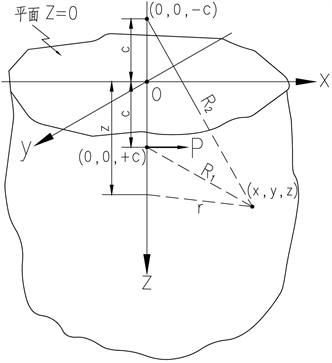Figure 1. Sketch of Mindlin model

$\begin{array}{c}{\sigma }_{x}=\frac{Px}{8\pi \left(1-\nu \right)}\left\{\frac{1-2\nu }{{R}_{1}^{3}}+\frac{3{x}^{2}}{{R}_{1}^{5}}-\frac{\left(1-2\nu \right)\left(5-4\nu \right)}{{R}_{2}^{3}}+\frac{3\left(3-4\nu \right){x}^{2}}{{R}_{2}^{5}}\\ \text{\hspace{0.17em}}+\frac{4\left(1-\nu \right)\left(1-2\nu \right)}{{R}_{2}{\left({R}_{2}+z+c\right)}^{2}}\left[3-\frac{{x}^{2}\left(3{R}_{2}+z+c\right)}{{R}_{2}^{2}\left({R}_{2}+z+c\right)}\right]\\ \text{\hspace{0.17em}}-\frac{6c}{{R}_{2}^{5}}\left[3c-\left(3-2\nu \right)\left(z+c\right)+\frac{5{x}^{2}z}{{R}_{2}^{2}}\right]\right\}\end{array}$ (1)

$\begin{array}{c}{\sigma }_{y}=\frac{Px}{8\pi \left(1-\nu \right)}\left\{-\frac{1-2\nu }{{R}_{1}^{3}}+\frac{3{y}^{2}}{{R}_{1}^{5}}-\frac{\left(1-2\nu \right)\left(3-4\nu \right)}{{R}_{2}^{3}}+\frac{3\left(3-4\nu \right){y}^{2}}{{R}_{2}^{5}}\\ \text{\hspace{0.17em}}+\frac{4\left(1-\nu \right)\left(1-2\nu \right)}{{R}_{2}{\left({R}_{2}+z+c\right)}^{2}}\left[1-\frac{{y}^{2}\left(3{R}_{2}+z+c\right)}{{R}_{2}^{2}\left({R}_{2}+z+c\right)}\right]\\ \text{\hspace{0.17em}}-\frac{6c}{{R}_{2}^{5}}\left[c-\left(1-2\nu \right)\left(z+c\right)+\frac{5y{z}^{2}}{{R}_{2}^{2}}\right]\right\}\end{array}$ (2)

$\begin{array}{c}{\sigma }_{z}=\frac{Px}{8\pi \left(1-\nu \right)}\left\{-\frac{1-2\nu }{{R}_{1}^{3}}+\frac{3{\left(z-c\right)}^{2}}{{R}_{1}^{5}}-\frac{1-2\nu }{{R}_{2}^{3}}+\frac{3\left(3-4\nu \right){\left(z+c\right)}^{2}}{{R}_{2}^{5}}\\ \text{\hspace{0.17em}}-\frac{6c}{{R}_{2}^{5}}\left[c+\left(1-2\nu \right)\left(z+c\right)+\frac{5z{\left(z+c\right)}^{2}}{{R}_{2}^{2}}\right]\right\}\end{array}$ (3)

$\begin{array}{c}{\tau }_{xy}=\frac{Py}{8\pi \left(1-\nu \right)}\left\{\frac{1-2\nu }{{R}_{1}^{3}}+\frac{3{x}^{2}}{{R}_{1}^{5}}-\frac{1-2\nu }{{R}_{2}^{3}}+\frac{3\left(3-4\nu \right){x}^{2}}{{R}_{2}^{5}}+\frac{4\left(1-\nu \right)\left(1-2\nu \right)}{{R}_{2}{\left({R}_{2}+z+c\right)}^{2}}\\ \text{\hspace{0.17em}}+\left[1-\frac{{x}^{2}\left(3{R}_{2}+z+c\right)}{{R}_{2}^{2}\left({R}_{2}+z+c\right)}\right]-\frac{6cz}{{R}_{2}^{5}}\left[1-\frac{5{x}^{2}}{{R}_{2}^{2}}\right]\right\}\end{array}$ (4)

${\tau }_{yz}=\frac{Pxy}{8\pi \left(1-\nu \right)}\left\{\frac{3\left(z-c\right)}{{R}_{1}^{5}}+\frac{3\left(3-4\nu \right)\left(z+c\right)}{{R}_{2}^{5}}-\frac{6c}{{R}_{2}^{5}}\left[1-2\nu +\frac{5z\left(z+c\right)}{{R}_{2}^{2}}\right]\right\}$ (5)

$\begin{array}{l}{\tau }_{zx}=\frac{P}{8\pi \left(1-\nu \right)}\left\{\frac{\left(1-2\nu \right)\left(z-c\right)}{{R}_{1}^{3}}+\frac{3{x}^{3}\left(z-c\right)}{{R}_{1}^{5}}-\frac{\left(1-2\nu \right)\left(z-c\right)}{{R}_{2}^{3}}+\frac{3\left(3-\nu \right){x}^{2}\left(z+c\right)}{{R}_{2}^{5}}\\ \text{\hspace{0.17em}}\text{\hspace{0.17em}}\text{\hspace{0.17em}}\text{\hspace{0.17em}}\text{\hspace{0.17em}}\text{\hspace{0.17em}}\text{\hspace{0.17em}}+\frac{6c}{{R}_{2}^{5}}\left[z\left(z+c\right)-\left(1-2\nu \right){z}^{2}-\frac{5xz\left(z+c\right)}{{R}_{2}^{2}}\right]\right\}\end{array}$ (6)

$\begin{array}{l}{u}_{x}=\frac{P}{16\pi G\left(1-\nu \right)}\left\{\frac{3-4\nu }{{R}_{1}}+\frac{{x}^{2}}{{R}_{1}^{3}}+\frac{1}{{R}_{2}}+\frac{\left(3-4\nu \right){x}^{2}}{{R}_{2}^{3}}+\frac{2cz}{{R}_{2}^{3}}\left(1-\frac{3{x}^{2}}{{R}_{2}^{2}}\right)\\ \text{\hspace{0.17em}}\text{\hspace{0.17em}}\text{\hspace{0.17em}}\text{\hspace{0.17em}}\text{ }\text{ }\text{\hspace{0.17em}}+\frac{4\left(1-\nu \right)\left(1-2\nu \right)}{{R}_{2}+z+c}\left[1-\frac{{x}^{2}}{{R}_{2}\left({R}_{2}+z+c\right)}\right]\right\}\end{array}$ (7)

${u}_{y}=\frac{Pxy}{16\pi G\left(1-\nu \right)}\left\{\frac{1}{{R}_{1}^{3}}+\frac{3-4\nu }{{R}_{2}^{3}}-\frac{6cz}{{R}_{2}^{3}}-\frac{4\left(1-\nu \right)\left(1-2\nu \right)}{{R}_{2}{\left({R}_{2}+z+c\right)}^{2}}\right\}$ (8)

${u}_{z}=\frac{Px}{16\pi G\left(1-\nu \right)}\left\{\frac{z-c}{{R}_{1}^{3}}+\frac{\left(3-4\nu \right)\left(z-c\right)}{{R}_{2}^{3}}-\frac{6cz\left(z+c\right)}{{R}_{2}^{5}}+\frac{4\left(1-\nu \right)\left(1-2\nu \right)}{{R}_{2}\left({R}_{2}+z+c\right)}\right\}$ (9)

c——水平力作用点与土体表面的垂直距离(m)；

z——计算点与土体表面间的垂直距离(m)；

x——水平力作用点与计算点之间在X轴上的投影距离(m)；

P——水平集中力的大小(N)；

G——土体剪切模量(MPa)，可通过弹性模量E折算 $G=\frac{E}{2\left(1+\nu \right)}$

$\nu$ ——土体泊松比。

2.2. 三轴水泥土搅拌桩挤土效应的Mindlin理论解析

2.2.1. 简化假定

1) 所研究的土体为各向同性线弹性体；

2) 喷浆压力和沉桩钻进压力合并成单一荷载考虑；

3) 三轴搅拌桩横截面等效成外包圆截面，每次加载段长度固定，为d1

4) 假定搅拌桩施工过程荷载分段施加，位移场分段累加：前一阶段为后一阶段的初始位移场。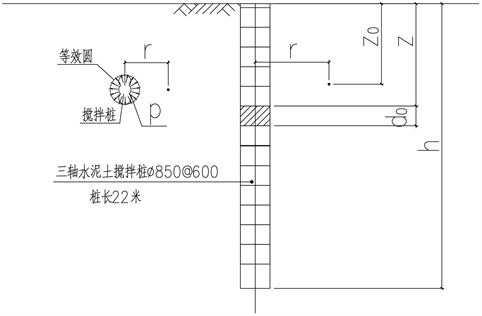Figure 2. Mindlin model sketch of TMCP

2.2.2. 单段加载时的位移公式

$r={\left({r}_{0}^{2}+{\left({r}_{0}+{r}_{1}\right)}^{2}-2\left({r}_{0}+{r}_{1}\right){r}_{0}\mathrm{cos}\theta \right)}^{\frac{1}{2}}$ (10)

${{R}^{\prime }}_{1}={\left[{r}_{0}^{2}+{\left({r}_{0}+{r}_{1}\right)}^{2}-2\left({r}_{0}+{r}_{1}\right){r}_{0}\mathrm{cos}\theta +{\left(z-c\right)}^{2}\right]}^{\frac{1}{2}}$ (11)

${{R}^{\prime }}_{2}={\left[{r}_{0}^{2}+{\left({r}_{0}+{r}_{1}\right)}^{2}-2\left({r}_{0}+{r}_{1}\right){r}_{0}\mathrm{cos}\theta +{\left(z+c\right)}^{2}\right]}^{\frac{1}{2}}$ (12)

$\text{d}P=p\cdot {d}_{1}\cdot r\cdot \text{d}\theta$ (13)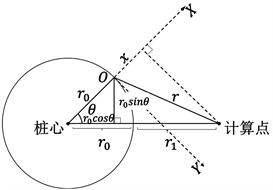Figure 3. Calculation sketch at the interval $\theta \in \left[-{90}^{\circ },{90}^{\circ }\right]$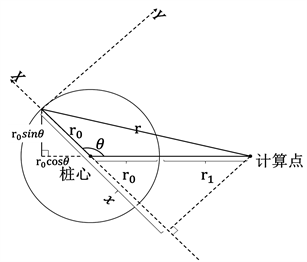Figure 4. Calculation sketch at the interval $\theta \in \left[{90}^{\circ },{270}^{\circ }\right]$

$\begin{array}{l}{U}_{x}\left(z\right)=\frac{p\cdot {d}_{1}\cdot r}{16\pi G\left(1-\nu \right)}{\int }_{0}^{2\pi }\left\{\frac{3-4\nu }{{{R}^{\prime }}_{1}}+\frac{{\left[\left({r}_{0}+{r}_{1}\right)\mathrm{cos}\theta -{r}_{0}\right]}^{2}}{{{R}^{\prime }}_{1}^{3}}+\frac{1}{{{R}^{\prime }}_{2}}+\frac{\left(3-4\nu \right){\left[\left({r}_{0}+{r}_{1}\right)\mathrm{cos}\theta -{r}_{0}\right]}^{2}}{{{R}^{\prime }}_{2}^{3}}\\ \text{\hspace{0.17em}}\text{\hspace{0.17em}}\text{\hspace{0.17em}}\text{\hspace{0.17em}}\text{\hspace{0.17em}}\text{\hspace{0.17em}}\text{\hspace{0.17em}}\text{\hspace{0.17em}}\text{\hspace{0.17em}}\text{\hspace{0.17em}}\text{\hspace{0.17em}}+\frac{2cz}{{{R}^{\prime }}_{2}^{3}}\left(1-\frac{3{\left[\left({r}_{0}+{r}_{1}\right)\mathrm{cos}\theta -{r}_{0}\right]}^{2}}{{{R}^{\prime }}_{2}^{2}}\right)+\frac{4\left(1-\nu \right)\left(1-2\nu \right)}{{{R}^{\prime }}_{2}+z+c}\left[1-\frac{{\left[\left({r}_{0}+{r}_{1}\right)\mathrm{cos}\theta -{r}_{0}\right]}^{2}}{{{R}^{\prime }}_{2}\left({{R}^{\prime }}_{2}+z+c\right)}\right]\right\}d\theta \end{array}$ (14)

$\begin{array}{l}{U}_{z}\left(z\right)=\frac{p\cdot {d}_{1}\cdot r}{16\pi G\left(1-\nu \right)}{\int }_{0}^{2\pi }\left[\left({r}_{0}+{r}_{1}\right)\mathrm{cos}\theta -{r}_{0}\right]\\ \text{\hspace{0.17em}}\text{\hspace{0.17em}}\text{\hspace{0.17em}}\text{\hspace{0.17em}}\text{\hspace{0.17em}}\text{\hspace{0.17em}}\text{\hspace{0.17em}}\text{\hspace{0.17em}}\text{\hspace{0.17em}}\text{\hspace{0.17em}}\text{\hspace{0.17em}}\text{\hspace{0.17em}}\cdot \left\{\frac{z-c}{{{R}^{\prime }}_{1}^{3}}+\frac{\left(3-4\nu \right)\left(z-c\right)}{{{R}^{\prime }}_{2}^{3}}-\frac{6cz\left(z+c\right)}{{{R}^{\prime }}_{2}^{5}}+\frac{4\left(1-\nu \right)\left(1-2\nu \right)}{{{R}^{\prime }}_{2}\left({{R}^{\prime }}_{2}+z+c\right)}\right\}\text{d}\theta \end{array}$ (15)

p——搅拌桩的桩头荷载集度(Pa)。

2.2.3. 整桩施工时的位移公式

${u}_{total}={u}_{1}+{u}_{2}+{u}_{3}+\cdots +{u}_{i}+\cdots +{u}_{n}$ (16)

n——划分的小阶段数目。

3. 案例分析

3.1. 工程概况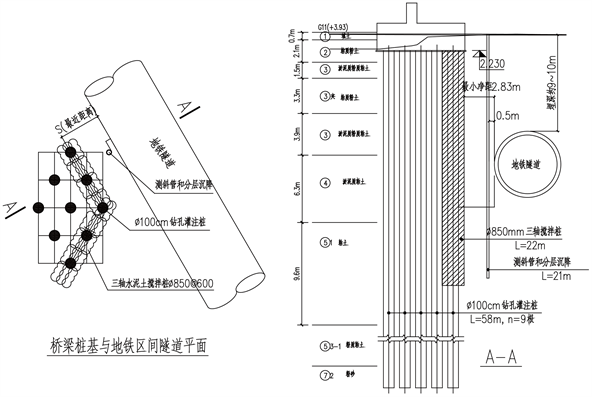Figure 5. Subway tunnel and pile foundation of bridge

3.2. 地质参数Table 1. Properties of soils for Mindlin equation

3.3. 现场量测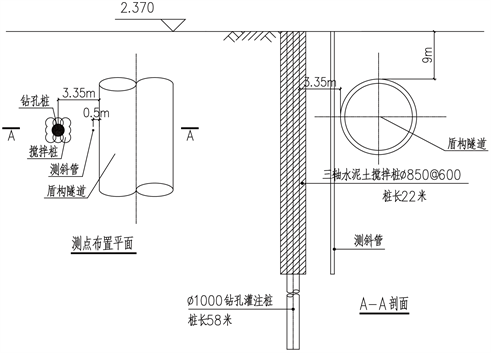Figure 6. Layout of inclinometer casing

3.4. 计算结果及其分析

3.4.1. 搅拌桩施工导致邻近土体的水平位移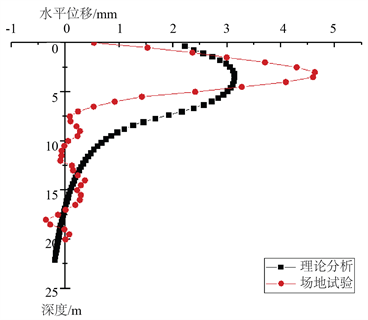Figure 7. Horizontal displacement of surrounding soil induced to TMCP driving

3.4.2. 搅拌桩施工导致邻近土体的竖向位移Figure 8. Vertical displacement of surrounding soil induced to TMCP driving

3.4.3. 计算结果讨论

Mindlin基本解是在假定土体为各向同性的线弹性体基础上获得的，其解答适合于小变形情况。实际上土体是各向异性的弹塑性体，因此采用Mindlin公式得到的计算结果是近似的。

4. 结论

1) 搅拌桩施工时的挤土效应本质上就是施工过程中在大地半无限体内某一区域作用了分布荷载，因此，可以采用Mindlin解来预测邻近土体的位移；

2) 三轴搅拌桩的荷载效应计算时假定在其外包等效圆圆周上作用了径向分布力p，然后依此对Mindlin公式进行积分得到搅拌桩施工全过程中邻近土体的位移；

3) 尽管Mindlin解是在各向同性线弹性体假定基础上得到的，它仍可以真实反映搅拌桩施工时注浆压力及沉桩速度与挤土效应(及邻近土体的位移)的关系，可以帮助现场工程师快速获得施工参数控制的关键信息，这是采用复杂的三维有限元数值计算软件难以做到的。

2019年上海浦东新区城建系统科学技术研究项目，项目编号：2019-015。

 Mindlin, R.D. (1936) Force at a Point in the Interior of a Semi Infinite Solid. Physics, 7, 195-202.
https://doi.org/10.1063/1.1745385

 魏纲, 徐日庆. 软土隧道盾构法施工引起的纵向地面变形预测[J]. 岩土工程学报, 2005, 27(9): 1077-1081.

 许有俊, 张治华, 史明, 等. 土压平衡矩形顶管正面附加推力对地表隆起变形影响研究[J]. 西安建筑科技大学学报(自然科学版), 2019, 51(6): 814-824.

 秦世伟, 周艳坤, 莫泷. 静压桩沉桩施工对临近隧道的影响[J]. 上海大学学报(自然科学版), 2013, 19(5): 527-533.

 谷冠思. 桩基施工全过程对临近地铁隧道土体扰动行为研究[D]: [硕士学位论文], 上海: 同济大学, 2019.

 龚灵迪, 周萌, 赖允瑾, 谷冠思. 三轴搅拌桩施工挤土效应的试验研究[J]. 土木工程, 2020, 9(9): 864-874.
https://doi.org/10.12677/HJCE.2020.99091

 汤新春, 张荣, 赖允瑾, 谷冠思. 搅拌桩和钻孔桩先后施工对邻近地铁隧道的影响分析[J]. 土木工程, 2020, 9(9): 853-863.
https://doi.org/10.12677/HJCE.2020.99090

 黄芹, 陈耀华, 赖允瑾. 邻近地铁隧道钻孔灌注桩套打施工的防坍孔力学机理及工程应用[J], 现代隧道技术, 2018, 55(S2): 419-425.

Top